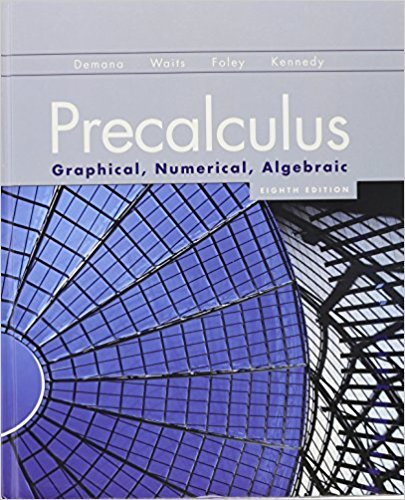×
×

# Solutions for Chapter 3: Exponential, Logistic, and Logarithmic Functions## Full solutions for Precalculus: Graphical, Numerical, Algebraic | 8th Edition

ISBN: 9780321656933Solutions for Chapter 3: Exponential, Logistic, and Logarithmic Functions

Solutions for Chapter 3
4 5 0 336 Reviews
25
5
##### ISBN: 9780321656933

This expansive textbook survival guide covers the following chapters and their solutions. Since 110 problems in chapter 3: Exponential, Logistic, and Logarithmic Functions have been answered, more than 43193 students have viewed full step-by-step solutions from this chapter. Chapter 3: Exponential, Logistic, and Logarithmic Functions includes 110 full step-by-step solutions. Precalculus: Graphical, Numerical, Algebraic was written by and is associated to the ISBN: 9780321656933. This textbook survival guide was created for the textbook: Precalculus: Graphical, Numerical, Algebraic, edition: 8th Edition.

Key Calculus Terms and definitions covered in this textbook
• Absolute value of a real number

Denoted by |a|, represents the number a or the positive number -a if a < 0.

• Anchor

See Mathematical induction.

• Circle graph

A circular graphical display of categorical data

• Exponential growth function

Growth modeled by ƒ(x) = a ? b a > 0, b > 1 .

• Horizontal component

See Component form of a vector.

• Increasing on an interval

A function ƒ is increasing on an interval I if, for any two points in I, a positive change in x results in a positive change in.

• Interval notation

Notation used to specify intervals, pp. 4, 5.

See Polynomial function in x.

• Multiplicative inverse of a complex number

The reciprocal of a + bi, or 1 a + bi = a a2 + b2- ba2 + b2 i

• Parametric equations

Equations of the form x = ƒ(t) and y = g(t) for all t in an interval I. The variable t is the parameter and I is the parameter interval.

• Positive numbers

Real numbers shown to the right of the origin on a number line.

• Power-reducing identity

A trigonometric identity that reduces the power to which the trigonometric functions are raised.

• Range (in statistics)

The difference between the greatest and least values in a data set.

• Re-expression of data

A transformation of a data set.

• Second

Angle measure equal to 1/60 of a minute.

• Symmetric property of equality

If a = b, then b = a

• System

A set of equations or inequalities.

• Unit vector

Vector of length 1.

• Vector

An ordered pair <a, b> of real numbers in the plane, or an ordered triple <a, b, c> of real numbers in space. A vector has both magnitude and direction.

• Vertex of a cone

See Right circular cone.

×